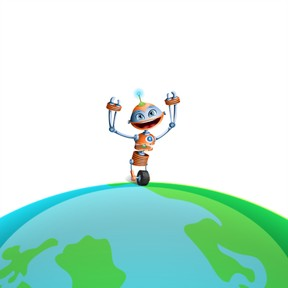Introduction to gravity

# Introduction to gravity

I can explain how objects' masses and distance are related.8,000 schools use Gynzy92,000 teachers use Gynzy1,600,000 students use Gynzy

## General

In this lesson, students will learn about Earth's gravitational force.

NGSS:
MS-PS2-2
MS-PS2-4

## Learning objective

Students will be able to explain how objects' masses and distance are related to their gravitational force.

## Introduction

Warm up with a problem about weight on the moon compared to weight on Earth.

## Instruction

Introduce the definition of gravity in the lesson. Discuss how all objects have gravity and mass. Bring up the scenario from the introduction and explain that although Virko the robot's weight stayed changed when he traveled from the Earth to the moon, his mass stayed the same. There will be practice problems to do as a class.

Next, discuss mass vs. gravity, as well as small and large gravitational forces. Students will talk with a partner about why Virko doesn't suddenly fly off the Earth of the sun is much more massive than Earth?

## Quiz

Students will respond to ten multiple-choice and fill-in-the-blank questions.

## Closing

Students will complete an exit slip that includes these questions:
1. Consider the picture below. What are the forces acting on the child (for this question, assume the child isn't moving)?
2. What is the difference between weight and mass?

### The online teaching platform for interactive whiteboards and displays in schools

• Save time building lessons

• Manage the classroom more efficiently

• Increase student engagement